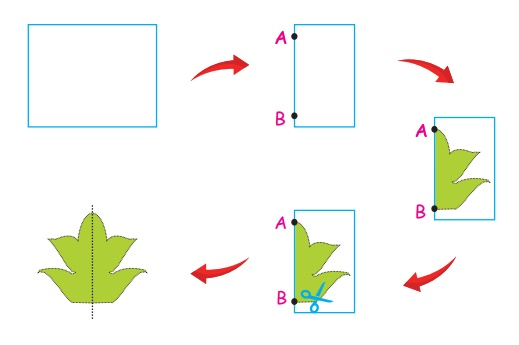Home | | Maths 4th Std | Introduction of symmetry

# Introduction of symmetry

In our day to day activity we see many leaves of plants, blades, wings of butterflies etc.

Introduction of symmetry

In our day to day activity we see many leaves of plants, blades, wings of butterflies etc.

The figures which can be divided into two parts of equal shape and size are called symmetrical figures.

EXAMPLE

The two halves of the figures are the mirror images of each other.EXAMPLEActivity

Draw the mirror symmetry using the other half.Symmetry

The line which divides a figure into two equal halves is called the line of symmetry.

Symmetry in Geometrical shapesA square has four lines of symmetry

A rectangle has two lines of symmetry.

A circle has infinitelines of symmetry

An equilateral triangle has three lines of symmetry

Activity

Find out the symmetrical figure and colour it:1. Able to explore intuitively the reflections through mirror ink blots, paper cutting and paper folding:

Activity

Take a piece of paper. Fold it exactly into half. Now take the folded edge and fold it into itself. Cut from one folded edge to the other folded edge. Open the paper and get a symmetrical figure.Activity

Let us understand the concept of symmetry by paper folding:

Take a white sheet of paper and fold it once such that it is divided into two equal parts.

Mark any two points say A and B on the folded line. As shown in figure.

Draw a closed figure on the folded paper beginning at a point and ending at the other point.

Cut the closed figure along the dotted lines marked.

Unfold the paper. The half shapes on the either side of the line of fold AB are exactly the same.

The line of the fold is the line of symmetry.2. Able to draw top view, front view and side view of simple objects.3. To observe from the surroundings and from day to day life situations and identify symmetrical objects.

Activity

Check whether the following pictures are symmetrical or not symmetrical4. Collects record symmetrical objects whenever / wherever they see.

Activity

Observe the objects around you at home. List the name and shape of the objects. Above these objects which can be called as symmetrical.

Name : Shape

1. Clock - Circle

2. Television - Rectangle

3. Funnel - Cone

4. Books - Rectangle

5. Match boxes - Rectangle

6. Carom board - Square

7. Orange - Sphere

5. To draw such symmetrical objects and naming the same.Tags : Geometry | Term 2 Chapter 1 | 4th Maths , 4th Maths : Term 2 Unit 1 : Geometry
Study Material, Lecturing Notes, Assignment, Reference, Wiki description explanation, brief detail
4th Maths : Term 2 Unit 1 : Geometry : Introduction of symmetry | Geometry | Term 2 Chapter 1 | 4th Maths